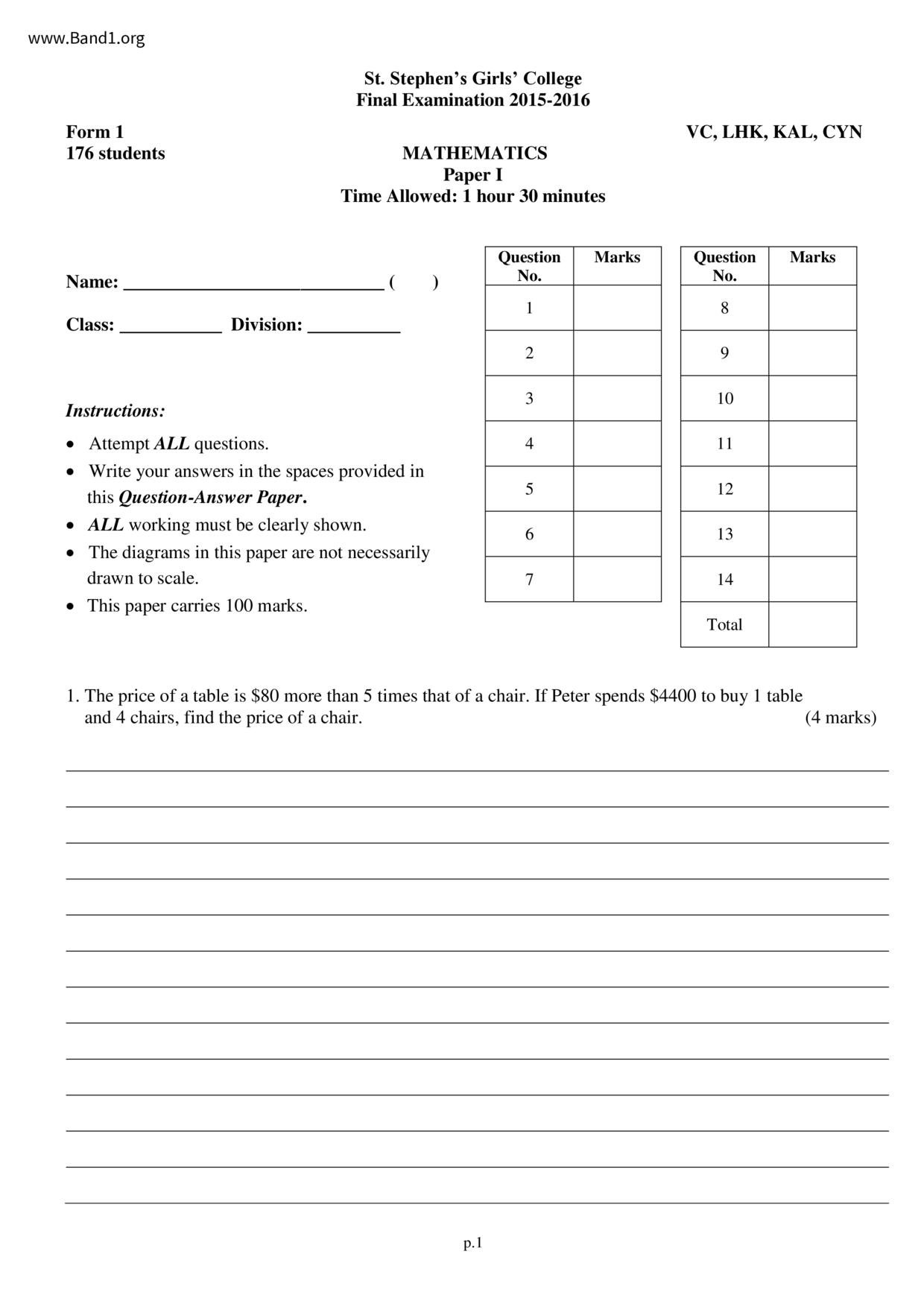# 中一 數學試卷 (F1 Maths Past Paper)

6934

pdf

12

-Ma-P1-15-16-fy

▼ 圖片只作預覽, 如欲下載整份卷, 請按「免費成為會員」 ▼▲ 圖片只作預覽, 如欲下載整份卷, 請按「免費成為會員」 ▲

## 中一數學試卷 PDF 下載

176 students
St. Stephen's Girls' College
Final Examination 2015-2016
MATHEMATICS
Time Allowed: 1 hour 30 minutes
Instructions:
• Attempt ALL questions.
• ALL working must be clearly shown.
• The diagrams in this paper are not necessarily
drawn to scale.
• This paper carries 100 marks.
VC, LHK, KAL, CYN
Question Marks
1. The price of a table is \$80 more than 5 times that of a chair. If Peter spends \$4400 to buy 1 table
and 4 chairs, find the price of a chair.
F.1 Mathematics Paper I Final Examination 2015-2016
12. In the figure, APC and DPB are straight lines. It is given that
DA // CB and DA = CB.
(b) Find the value of x.
F.1 Mathematics Paper I Final Examination 2015-2016
13. In the figure, AEC is a straight line.
It is given that ZABC =ZCDE, AE = 4, ED = 6,
DC-9, BC= 8, AB = 12 and EC = x.
(a) Prove that AABC~ACDE.
(b) Find the value of x.
F.1 Mathematics Paper I Final Examination 2015-2016
14. The figure shows AABC in a rectangular coordinate plane.
(a) In the figure, draw the image after each of the following transformations.
(All the vertices of the images drawn must be labeled accordingly.)
(i) AABC is translated 1 unit to the right and then 2 units downwards to get AA₁B₁C₁.
(ii) AA₁B₁C₁ is reflected in the straight line L to get AA2B₂C2.
(iii) AA₁B₁C₁ is rotated through 90° in the clockwise direction about the origin O to get AA3B3C3.
(b) Actually we can get AA3B3C3 from AA2B2C2 through a single transformation. Describe the
* End of Paper *
F.1 Mathematics Paper I Final Examination 2015-2016
2. It is given that the general term of a sequence is 32 - 3n.
(a) Find the 4th term and the 5th term of the sequence.
(b) Determine whether -4 is a term of the sequence. Explain your answer.
3. Ming is going to cover his reading room with wall paper. The reading room is 5 m long, 4 m wide and
3 m high. The total area of the room's door and windows is 8 m². If one roll of wallpaper can cover
6 m², find the least number of rolls of wallpaper needed to cover the walls of Ming's reading room.
F.1 Mathematics Paper I Final Examination 2015-2016
4. The length and the width of a rectangle are k cm and (k-10) cm respectively. It is known that the
perimeter of the rectangle is not less than 160 cm.
(a) Set up an inequality to represent the above situation.
(b) Is it possible that the length of the rectangle is 45 cm? Explain your answer.
(c) If the perimeter of the rectangle is 216 cm, find its length.
F.1 Mathematics Paper I Final Examination 2015-2016
5. The following frequency distribution table shows the weights of 36 students in a class.
Weight (kg)
(a) Find the value of x.
(b) Find the percentage of students with weights 49.5 kg or above.
(c) If a pie chart is used to present the above data, find the angle of the sector representing
'35kg - 39kg'.
F.1 Mathematics Paper I Final Examination 2015-2016
6. The broken-line graph shows the sales of a company from 2011 to 2015.
Sales of a company
(million dollars)
2011 2012 2013 2014 2015
Between which two years did the sales of the company decrease? Find the decrease in the sales
of the company.
Find the percentage increase of the sales of the company from 2013 to 2015.
F.1 Mathematics Paper I Final Examination 2015-2016
7. (a) Find the value of x.
(b) Find the value of ZAOC.
The figure shows a polar coordinate plane.
110° 100° 90°
260 270 280⁰ 290⁰ 00⁰
1 2 3 4 5 6
(a) Plot the points A(3, 60°), B(4, 150°) and C(5, 240°) in the figure.
(c) Find the area of AABC.
300⁰ - 2x
F.1 Mathematics Paper I Final Examination 2015-2016
9. In the figure, the coordinates of P and Q are (-6, 4) and (6, 3) respectively. P is translated downwards
by 10 units to a point S and Q is rotated clockwise about O through 90° to a point R.
(a) Mark R and S in the figure. Write down the coordinates of R and S.
(b) Let T be a point in quadrant IV such that QT LTS.
(i) Mark T' in the figure and write down the coordinates of T.
(ii) Find the area of trapezium PQTS.
(c) By using the result of (b)(ii), or otherwise, find the area of quadrilateral PQRS.
(a) The coordinates of R are (
The coordinates of S are (
(b) (i) The coordinates of T are (
F.1 Mathematics Paper I Final Examination 2015-2016
10. In the figure, BEC is a straight line and FE // DC.
(a) Find the values of w, x, y and z.